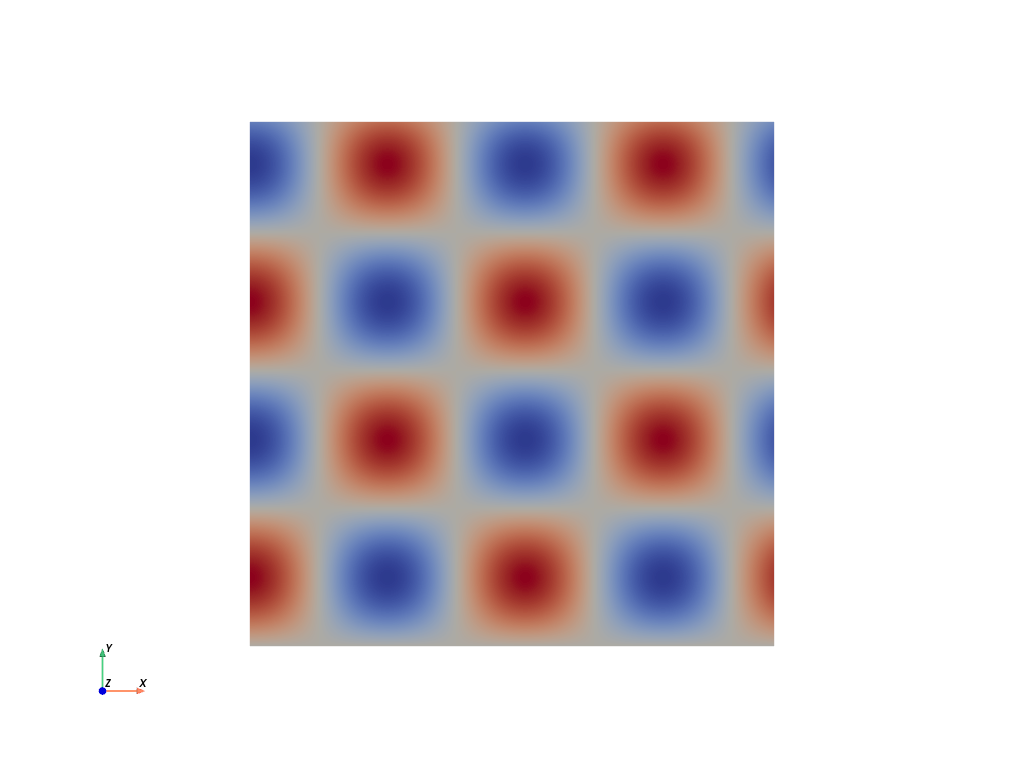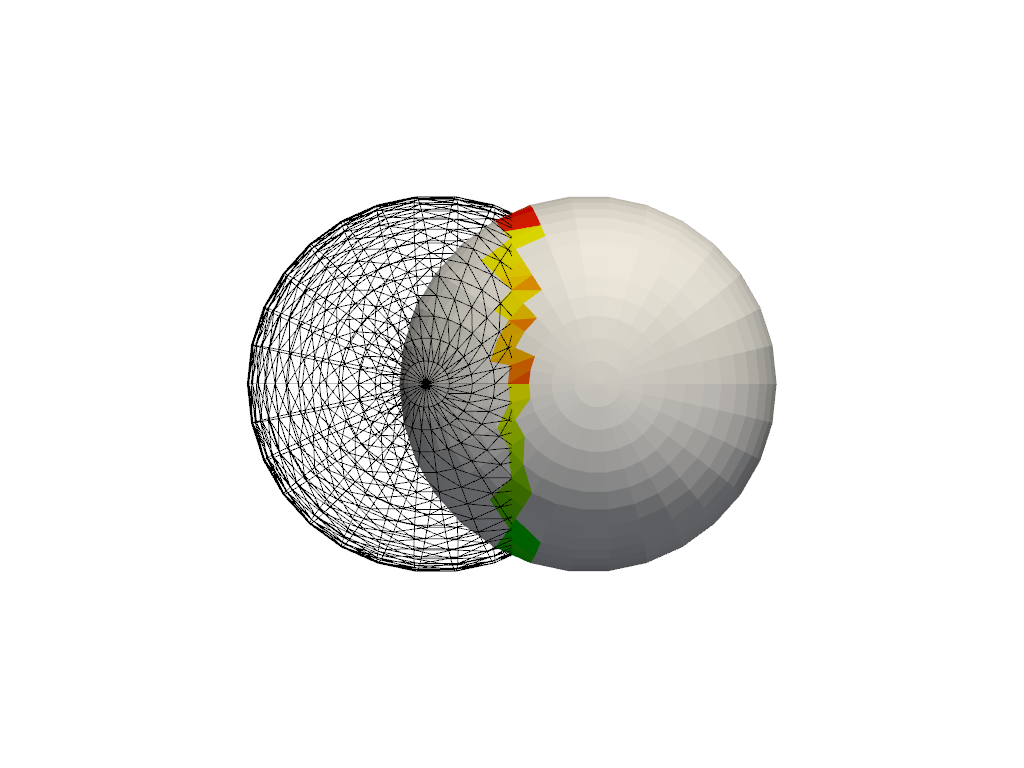# Transitioning from VTK to PyVista#

VTK is primarily developed in C++ and uses chained setter and getter commands to access data. Instead, PyVista wraps the VTK data types into numpy arrays so that users can benefit from its bracket syntax and fancy indexing. This section demonstrates the difference between the two approaches in a series of examples.

For example, to hard-code values for a vtk.vtkImageData data structure using VTK Python’s bindings, one would write the following:

```from math import cos, sin

import vtk
```

Create values for a 300x300 image dataset

In our example, we want to have values from the function

```127.5 + (1.0 + sin(x/25.0)*cos(y/25.0))
```
```values = vtk.vtkDoubleArray()
values.SetName("values")
values.SetNumberOfComponents(1)
values.SetNumberOfTuples(300 * 300)

for x in range(300):
for y in range(300):
values.SetValue(x * 300 + y, 127.5 + (1.0 + sin(x / 25.0) * cos(y / 25.0)))
```

Create the image structure

```image_data = vtk.vtkImageData()
image_data.SetOrigin(0, 0, 0)
image_data.SetSpacing(1, 1, 1)
image_data.SetDimensions(300, 300, 1)
```

Assign the values to the image

```image_data.GetPointData().SetScalars(values)
```
```0
```

As you can see, there is quite a bit of boilerplate that goes into the creation of a simple vtk.vtkImageData dataset. PyVista provides much more concise syntax that is more “Pythonic”. The equivalent code in PyVista is:

```import numpy as np
import pyvista as pv
```

Use the meshgrid function to create 2D “grids” of the x and y values. This section effectively replaces the vtkDoubleArray.

```xi = np.arange(300)
x, y = np.meshgrid(xi, xi)
values = 127.5 + (1.0 + np.sin(x / 25.0) * np.cos(y / 25.0))
```

Create the grid. Note how the values must use Fortran ordering.

```grid = pv.UniformGrid(dims=(300, 300, 1))
grid.point_data["values"] = values.flatten(order="F")
```
```/home/runner/.virtualenvs/.venv/lib/python3.9/site-packages/pyvista/core/grid.py:508: PyVistaDeprecationWarning: `dims` argument is deprecated. Please use `dimensions`.
warnings.warn(
```

Here, PyVista has done several things for us:

1. PyVista combines the dimensionality of the data (in the shape of the `numpy.ndarray`) with the values of the data in one line. VTK uses “tuples” to describe the shape of the data (where it sits in space) and “components” to describe the type of data (1 = scalars/scalar fields, 2 = vectors/vector fields, n = tensors/tensor fields). Here, shape and values are stored concretely in one variable.

2. `pyvista.UniformGrid` wraps vtk.vtkImageData, just with a different name; they are both containers of evenly spaced points. Your data does not have to be an “image” to use it with vtk.vtkImageData; rather, like images, values in the dataset are evenly spaced apart like pixels in an image.

Furthermore, since we know the container is for uniformly spaced data, pyvista sets the origin and spacing by default to `(0, 0, 0)` and `(1, 1, 1)`. This is another great thing about PyVista and Python! Rather than having to know everything about the VTK library up front, you can get started very easily! Once you get more familiar with it and need to do something more complex, you can dive deeper. For example, changing the origin and spacing is as simple as:

```grid.origin = (10, 20, 10)
grid.spacing = (2, 3, 5)
```
3. The name for the `point_array` is given directly in dictionary-style fashion. Also, since VTK stores data on the heap (linear segments of RAM; a C++ concept), the data must be flattened and put in Fortran ordering (which controls how multidimensional data is laid out in physically 1d memory; numpy uses “C”-style memory layout by default). This is why in our earlier example, the first argument to `SetValue()` was written as `x*300 + y`. Here, numpy takes care of this for us quite nicely and it’s made more explicit in the code, following the Python best practice of “Explicit is better than implicit”.

Finally, with PyVista, each geometry class contains methods that allow you to immediately plot the mesh without also setting up the plot. For example, in VTK you would have to do:

```actor = vtk.vtkImageActor()
actor.GetMapper().SetInputData(image_data)
ren = vtk.vtkRenderer()
renWin = vtk.vtkRenderWindow()
iren = vtk.vtkRenderWindowInteractor()
iren.SetRenderWindow(renWin)
iren.Initialize()
renWin.Render()
iren.Start()
```

However, with PyVista you only need:

```grid.plot(cpos='xy', show_scalar_bar=False, cmap='coolwarm')
```## PointSet Construction#

PyVista heavily relies on NumPy to efficiently allocate and access VTK’s C arrays. For example, to create an array of points within VTK one would normally loop through all the points of a list and supply that to a vtkPoints class. For example:

```vtk_array = vtk.vtkDoubleArray()
vtk_array.SetNumberOfComponents(3)
vtk_array.SetNumberOfValues(9)
vtk_array.SetValue(0, 0)
vtk_array.SetValue(1, 0)
vtk_array.SetValue(2, 0)
vtk_array.SetValue(3, 1)
vtk_array.SetValue(4, 0)
vtk_array.SetValue(5, 0)
vtk_array.SetValue(6, 0.5)
vtk_array.SetValue(7, 0.667)
vtk_array.SetValue(8, 0)
vtk_points = vtk.vtkPoints()
vtk_points.SetData(vtk_array)
print(vtk_points)
```
```vtkPoints (0x56412f7b42b0)
Debug: Off
Modified Time: 13892809
Reference Count: 1
Registered Events: (none)
Data: 0x56412f7158e0
Data Array Name: Points
Number Of Points: 3
Bounds:
Xmin,Xmax: (0, 1)
Ymin,Ymax: (0, 0.667)
Zmin,Zmax: (0, 0)
```

To do the same within PyVista, you simply need to create a NumPy array:

```np_points = np.array([[0, 0, 0], [1, 0, 0], [0.5, 0.667, 0]])
```

Note

You can use `pyvista.vtk_points()` to construct a vtkPoints object, but this is unnecessary in almost all situations.

Since the end goal is to construct a `pyvista.DataSet`, you would simply pass the `np_points` array to the `pyvista.PolyData` constructor:

```poly_data = pv.PolyData(np_points)
```

Whereas in VTK you would have to do:

```vtk_poly_data = vtk.vtkPolyData()
vtk_poly_data.SetPoints(vtk_points)
```

The same goes with assigning face or cell connectivity/topology. With VTK you would normally have to loop using `InsertNextCell()` and `InsertCellPoint()`. For example, to create a single cell (triangle) and then assign it to vtkPolyData:

```cell_arr = vtk.vtkCellArray()
cell_arr.InsertNextCell(3)
cell_arr.InsertCellPoint(0)
cell_arr.InsertCellPoint(1)
cell_arr.InsertCellPoint(2)
vtk_poly_data.SetPolys(cell_arr)
```

In PyVista, we can assign this directly in the constructor and then access it (or change it) from the `faces` attribute.

```faces = np.array([3, 0, 1, 2])
poly_data = pv.PolyData(np_points, faces)
poly_data.faces
```
```array([3, 0, 1, 2])
```

While most features can, not everything can be simplified in PyVista without losing functionality or performance.

In the `collision` filter, we demonstrate how to calculate the collision between two meshes. For example:

```# create a default sphere and a shifted sphere
mesh_a = pv.Sphere()
mesh_b = pv.Sphere(center=(-0.4, 0, 0))
out, n_coll = mesh_a.collision(mesh_b, generate_scalars=True, contact_mode=2)
```
```pl = pv.Plotter()